# Functions

## Functions | Why should we learn Functions

In this article, we will learn about functions, we will see what are functions, then we will see what is parameter passing and what are the different methods of passing parameters like pass by value, pass by address and pass by reference. In C language, we have only pass by value and pass by address, but in C++ we also have pass by reference. Please read our previous article, where we discussed how to access a structure using a pointer. We will take some simple examples and explain all these parameters passing, Let’s start with functions.

##### What are the Functions?

The function is a piece of code that performs a specific task. We have already studied structures. In structure, we studied that structure is a group of related data members. Now, the function is a group of related instructions that perform a specific task. So, grouping data members is a structure, grouping instructions is a function.

Functions are also called modules or procedures. Instead of writing a single main program i.e. writing everything inside the main function, we can break the main function into small manageable size pieces and we can separate the repeating tasks or smaller tasks as a function.

##### For example;

If we write one program, and if we put everything inside the main function, then such type of programming approach is called Monolithic programming. If your main function contains thousands of lines of code, then it is becoming very difficult to manage. This is actually not a good programming approach.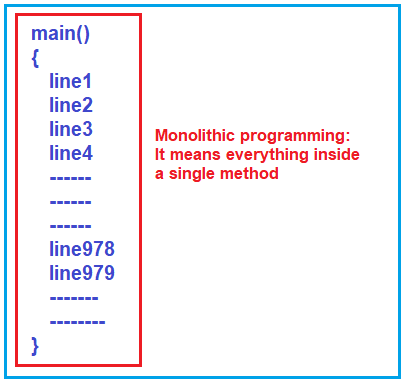So, if we broke the program into smaller tasks, i.e. into many smaller functions and each function performs a specific task, then such type of programming is called “modular programming” or “procedural programming” and this approach is good for developing.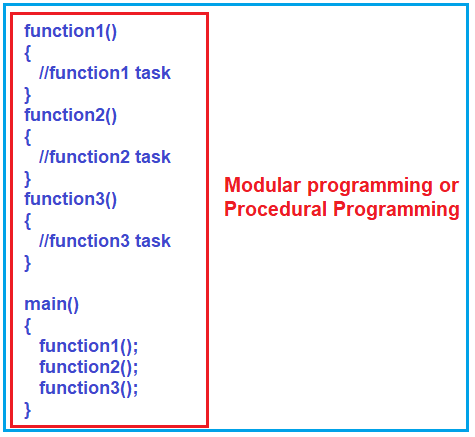As shown in the above image, the first function i.e. function1() will perform some specific task and another function i.e. function2() will performing some other task, and similarly, function3() may perform some task. So, in this way, we can break the larger task into smaller simple tasks, and then we can use them all together inside the main function.

Here, in the Modular Programming Approach, you can break the program into smaller tasks and you can focus on smaller tasks and finish them and make them perfect. It is easy for one single individual to develop the application even you can break this software project into a team of programmers where each programmer will focus on one or many smaller tasks.

This Modular style of programming approach has increased productivity and also reusability. For example, if you want the logic of function2 three times inside the main method, then you simply need to call function2 three times. That means we are reusing the logic defined in function 2. This is called reusability.

The same function can also be used in other software projects also. You can put the group of functions together in a library. These are the benefits of functions.

C language is a procedural or modular programming language and this is the topmost feature supported by C programming which is also there in C++. Beyond this one, C++ follows object-orientation where you group the related data members and functions together and put them in a single class.

##### Modular Programming Examples:

Now, let us focus on modular programming and let us see how we can break a program into functions. Let us write a simple program using a function and learn how we can separate the task from the main function.

In the below example, we have implemented the logic to add two numbers inside the main function only.

```#include <stdio.h>
int main ()
{
int x, y;
x = 10;
y = 5;
int z = x + y;
printf ("sum is %d", z);
}
```

As you can see in the above code, first we declare two variables x and y and then we initialize these two variables with values 10 and 5 respectively. Then we add two variables and store the result in another variable i.e. z and finally print the value of z in the console and that what you can see in the output.

Let us see how to write the same Program using Function. For better understanding please have a look at the below image.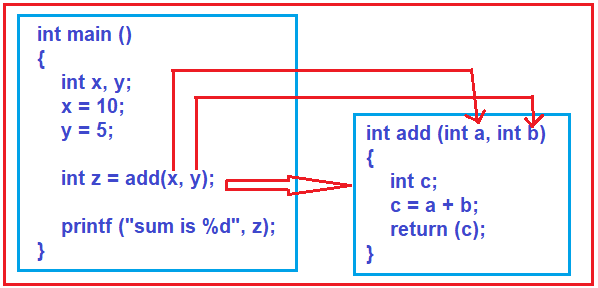As you can see in the above image, we created a function called add which takes two input parameters a and b of type integer. This add function adds the two integer numbers it received as input parameter and store the result in variable c and return that result.

Now see the main function. From the main function, we are calling the add function and while calling the add function we are passing two parameters i.e. x and y (actually we are passing the values stored in x and y) and these parameters’ values will go into a and b. The add function then adds these two values and returns the result to the calling function (the function which called the add method) i.e. the main method. The main method then store the result coming from the add method into the variable z and then print the result on the output window.

The complete code is given below.

```#include <stdio.h>
int add (int a, int b)
{
int c;
c = a + b;
return (c);
}

int main ()
{
int x, y;
x = 10;
y = 5;
int z = add (x, y);
printf ("sum is %d", z);
}
```
##### Different Parts of a Function:

To understand the different parts of a function, please have a look at the below image.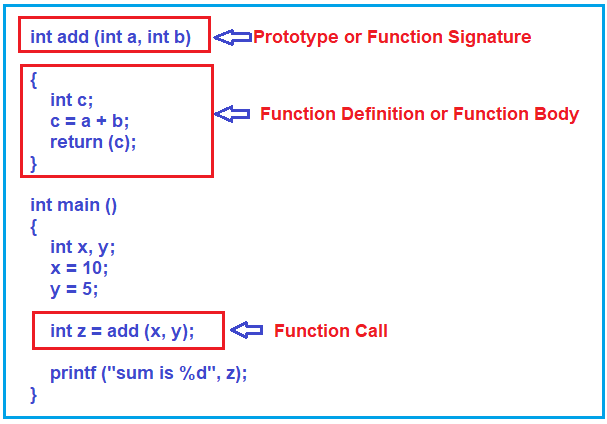##### What are the Parameters of a function?

For a better understanding of the function parameters, please have a look at the below image.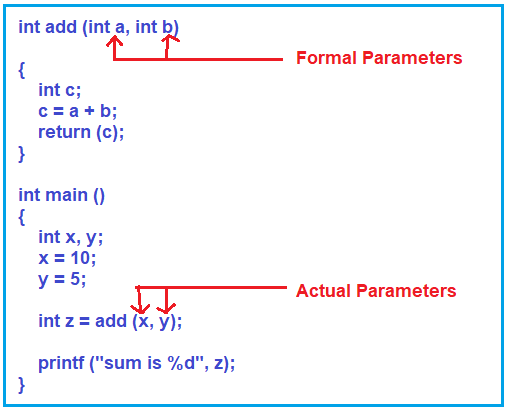As you can see in the above image, we are passing two values x and y to the add function which is taking two parameters (a and b). The parameters (x and y) which are passing to the add function are called Actual Parameters. The parameters (a and b) which are taken by the add method are called Formal Parameters. When we call the add method the values of actual parameters are copied to the formal parameters. So, x value i.e. 10 is copied to a, and y value i.e. 5 is copied to b.

##### How does it work inside the main memory?

When the program starts i.e. when the main method starts its execution, three variables (x, y, and z) are declared inside the stack, that is inside the activation area of the main function. Then the x and y are assigned with values 10 and 15 respectively. And then the main method calls the add method. Once the add method is called, its own activation area is created inside the stack and it will have its own variables i.e. variables a, b, and c are created inside this activation area. Then the value of x i.e. 10 and the value of y i.e. 5 passed to add function are copied to the variable a and b respectively. Then the add method adds the two numbers and the result is 15 which is stored in the variable c and that result i.e. 15 is returned from the add method. That result coming from the add method is stored in the variable z and that will be print in the console window. For better understanding, please have a look at the following image.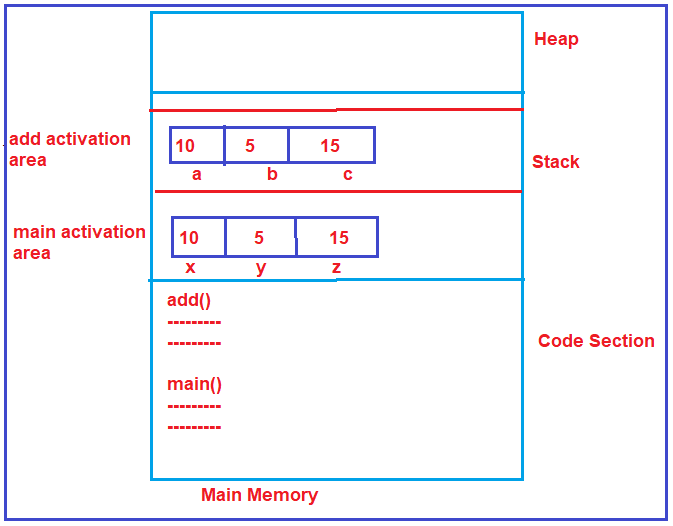So, this is what happens inside the main memory when we are writing functions. One more point you need to remember is, one function cannot access the variables of other functions.

In the next article, I am going to discuss the Parameter Passing Techniques in detail. Here, in this article, I try to explain Functions and I hope you enjoy this article.